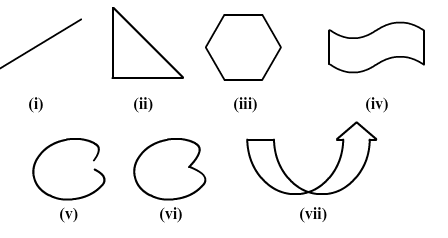×#### Thank you for registering.

One of our academic counsellors will contact you within 1 working day.

Click to Chat

1800-1023-196

+91-120-4616500

CART 0

• 0

MY CART (5)

Use Coupon: CART20 and get 20% off on all online Study Material

ITEM
DETAILS
MRP
DISCOUNT
FINAL PRICE
Total Price: Rs.

There are no items in this cart.
Continue Shopping• Complete JEE Main/Advanced Course and Test Series
• OFFERED PRICE: Rs. 15,900
• View Details

```Chapter 20: Mensuration Exercise 20.1

Question: 1

Which of the following are closed curves? Which of them are simple?Solution:

Figure (ii), (iii), (iv), (vi), and (vii) are closed curves, whereas Figure (ii), (iii), (iv) and (vi) are simple closed curves.

Question: 2

Define perimeter of a closed figure.

Solution:

The length of the boundary of a closed figure is known as its permieter.

Question: 3

Find the perimeter of each of the following shapes:Solution:

Perimeter = Sum of lengths of all sides os a closed figure

(i) Perimeter = ( 4 + 2 + 1 + 5) cm = 12 cm

(ii) Perimeter = (23 + 35 + 40 + 35) cm = 133 cm

(iii) Perimeter = (15 + 15 + 15 + 15) cm = 60 cm

(iv) Perimeter = (3 + 3 + 3 + 3 + 3) cm = 15 cm
```### Course Features

• 728 Video Lectures
• Revision Notes
• Previous Year Papers
• Mind Map
• Study Planner
• NCERT Solutions
• Discussion Forum
• Test paper with Video Solution